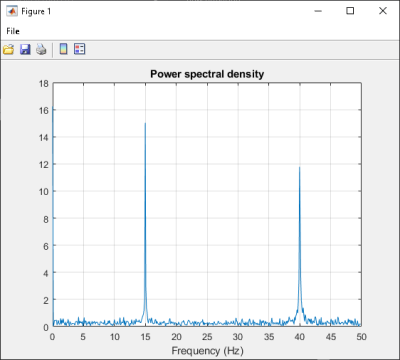## Use Multiple Classes in .NET Assembly

This example shows you how to create a .NET assembly that uses multiple classes to analyze a signal and graph the result.

In this example, you perform the following steps:

• Use the MATLAB® Compiler SDK™ product to create an assembly (`SpectraComp`) containing more than one class.

• Access the component in a C# application (`SpectraApp.cs`) or a Microsoft® Visual Basic® application (`SpectraApp.vb`), including use of the `MWArray` class hierarchy to represent data.

• Build and run the application using the Visual Studio® .NET development environment.

### SpectraComp Application

The class `SignalAnalyzer` performs a fast Fourier transform (FFT) on an input data array. A method of this class, `computefft`, returns the results of that FFT as two output arrays—an array of frequency points and the power spectral density.

The second class, `Plotter`, graphs the returned data using the `plotfft` method. The two methods `computefft` and `plotfft` encapsulate MATLAB functions. `computefft` computes the FFT and power spectral density of the input data and computes a vector of frequency points based on the length of the data entered and the sampling interval. `plotfft` plots the FFT data and the power spectral density in a MATLAB figure window.

### Files

 MATLAB Functions `computefft.m` `plotfft.m` MATLAB Function Location `matlabroot\toolbox\dotnetbuilder\Examples\VSVersion\NET\SpectraExample\SpectraComp\` C# Code Location `matlabroot\toolbox\dotnetbuilder\Examples\VSVersion\NET\SpectraExample\SpectraCSApp\SpectraApp.cs` Visual Basic Code Location `matlabroot\toolbox\dotnetbuilder\Examples\VSVersion\NET\SpectraExample\SpectraVBApp\SpectraApp.vb`

### Procedure

1. Copy the following folder that ships with the MATLAB product to your work folder:

```matlabroot\toolbox\dotnetbuilder\Examples\VSVersion\NET\SpectraExample ```

At the MATLAB command prompt, navigate to the new `SpectraExample\SpectraComp` subfolder in your work folder.

2. Examine the MATLAB functions `computefft.m` and `plotfft.m`.

3. Build the .NET component with the Library Compiler app or `compiler.build.dotNETAssembly`.

Name the library `SpectraComp`. Map a class named `SignalAnalyzer` to the function `computefft.m`, and map a second class named `Plotter` to the function `plotfft.m`.

For example, if you are using `compiler.build.dotNETAssembly`, type:

```cmap = containers.Map; cmap('SignalAnalyzer') = 'computefft.m'; cmap('Plotter') = 'plotfft'; buildResults = compiler.build.dotNETAssembly(cmap, ... 'AssemblyName','SpectraComp');```

For more details, see the instructions in Generate .NET Assembly and Build .NET Application.

4. Decide whether you are using C# or Visual Basic to access the component.

• C#

If you are using C#, write source code for a C# application that accesses the component.

The sample application for this example is in `SpectraExample\SpectraCSApp\SpectraApp.cs`.

The following statement shows how to use the `MWArray` class library to construct a `MWNumericArray` that is used as method input to the `computefft` function.

``````MWNumericArray data= new MWNumericArray(MWArrayComplexity.Real, MWNumericType.Double, numSamples);``````

The following statements create an instance of the class `SignalAnalyzer` and call the method `computefft`, requesting 3 outputs.

``````SignalAnalyzer signalAnalyzer = new SignalAnalyzer(); ... MWArray[] argsOut= signalAnalyzer.computefft(3, data, interval); ``````
• Visual Basic

If you are using Visual Basic, write source code for a Visual Basic application that accesses the component.

The sample application for this example is in `SpectraExample\SpectraVBApp\SpectraApp.vb`.

The following statements show how to use the `MWArray` class library to construct the necessary data types:

``````Dim data As MWNumericArray = New MWNumericArray_ (MWArrayComplexity.Real, MWNumericType.Double, numSamples) ... Dim resultArray As MWNumericArray = New MWNumericArray_ (MWArrayComplexity.Complex, MWNumericType.Double, numElements) ``````

The following statements create an instance of the class `SignalAnalyzer` and call the method `computefft`, requesting three outputs:

``````Dim signalAnalyzer As SignalAnalyzer = New SignalAnalyzer ... Dim argsOut() As MWArray = signalAnalyzer.computefft(3, data, MWArray.op_Implicit(interval))``````

In either case, the `SpectraApp` program does the following:

• Constructs an input array with values representing a random signal with two sinusoids at 15 and 40 Hz embedded inside of it

• Creates an `MWNumericArray` array that contains the data

• Instantiates a `SignalAnalyzer` object

• Calls the `computefft` method, which computes the FFT, frequency, and the spectral density

• Instantiates a `Plotter` object

• Calls the `plotfft` method, which plots the data

• Uses a `try`/`catch` block to handle exceptions

5. Open the .NET project file that corresponds to your application language using Visual Studio.

• C#

If you are using C#, the `SpectraCSApp` folder contains a Visual Studio .NET project file for this example. Open the project in Visual Studio .NET by double-clicking `SpectraCSApp.csproj` in Windows® Explorer. You can also open it from the desktop by right-clicking SpectraCSApp.csproj and selecting Open Outside MATLAB.

• Visual Basic

If you are using Visual Basic, the `SpectraVBApp` folder contains a Visual Studio .NET project file for this example. Open the project in Visual Studio .NET by double-clicking `SpectraVBApp.vbproj` in Windows Explorer. You can also open it from the desktop by right-clicking SpectraVBApp.vbproj and selecting Open Outside MATLAB.

6. Add a reference to your assembly file `SpectraComp.dll`.

7. Add a reference to the `MWArray` API.

 If MATLAB is installed on your system `matlabroot\toolbox\dotnetbuilder\bin\win64\\MWArray.dll` If MATLAB Runtime is installed on your system `\toolbox\dotnetbuilder\bin\win64\\MWArray.dll`

8. Build and run the `SpectraApp` application in Visual Studio .NET.

The program displays the following output:

```FFT: 1.0e+02 * 4.8646 + 0.0000i -0.0289 + 0.1080i -0.0326 + 0.0237i -0.0141 - 0.0148i 0.0674 - 0.0487i 0.0753 + 0.0669i 0.0275 - 0.0101i -0.0429 + 0.0472i 0.0803 - 0.1163i -0.0619 - 0.1072i 0.0565 - 0.0502i -0.0223 + 0.0587i -0.0853 - 0.0812i -0.0662 - 0.0143i 0.0543 - 0.0972i 0.0814 - 0.0463i -0.0981 - 0.0190i 0.0042 + 0.0083i -0.0339 + 0.0290i 0.0291 + 0.0036i Frequency: 0.0000 + 0.0000i 0.0999 + 0.0000i 0.1998 + 0.0000i 0.2997 + 0.0000i 0.3996 + 0.0000i 0.4995 + 0.0000i 0.5994 + 0.0000i 0.6993 + 0.0000i 0.7992 + 0.0000i 0.8991 + 0.0000i 0.9990 + 0.0000i 1.0989 + 0.0000i 1.1988 + 0.0000i 1.2987 + 0.0000i 1.3986 + 0.0000i 1.4985 + 0.0000i 1.5984 + 0.0000i 1.6983 + 0.0000i 1.7982 + 0.0000i 1.8981 + 0.0000i Power Spectral Density: 15.3755 + 0.0000i 0.3534 + 0.0000i 0.1274 + 0.0000i 0.0646 + 0.0000i 0.2628 + 0.0000i 0.3183 + 0.0000i 0.0925 + 0.0000i 0.2016 + 0.0000i 0.4465 + 0.0000i 0.3912 + 0.0000i 0.2387 + 0.0000i 0.1985 + 0.0000i 0.3723 + 0.0000i 0.2140 + 0.0000i 0.3520 + 0.0000i 0.2960 + 0.0000i 0.3158 + 0.0000i 0.0294 + 0.0000i 0.1411 + 0.0000i 0.0927 + 0.0000i Plotting Power spectral density, please wait...```## Related Examples